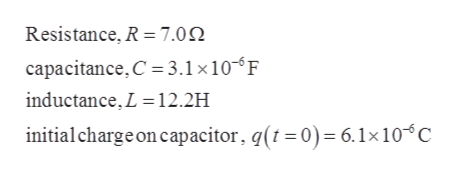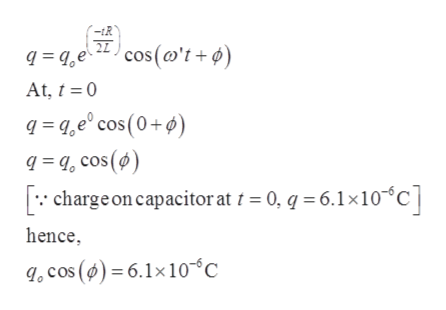# A single-loop circuit consists of a 7.0 Ω resistor, 12.2 H inductor, and a 3.1 μF capacitor. Initially the capacitor has a charge of 6.1 μC and the current is zero. Calculate the charge onthe capacitor N complete cycles later for (a) N = 5, (b) N =10, and (c) N = 100.

Question
93 views

A single-loop circuit consists of a 7.0 Ω resistor, 12.2 H inductor, and a 3.1 μF capacitor. Initially the capacitor has a charge of 6.1 μC and the current is zero. Calculate the charge on
the capacitor N complete cycles later for (a) N = 5, (b) N =10, and (c) N = 100.

check_circle

Step 1

Given,help_outlineImage TranscriptioncloseResistance, R = 7.0 capacitance, C 3.1 x10F inductance, L 12.2H initial charge on capacitor, q(t =0) = 6.1x10 C fullscreen
Step 2

In this LCR oscillation, Charge flow in the circuit is a function of time.help_outlineImage Transcriptionclose'-tR qqe2cos('t + ¢ At, t 0 qae° cos (0+ qa, cos() charge on capacitor at / = 0, q = 6.1x10 C hence .cos () = 6.1x10-'C fullscreen
Step 3

Angular frequency of LC...

### Want to see the full answer?

See Solution

#### Want to see this answer and more?

Solutions are written by subject experts who are available 24/7. Questions are typically answered within 1 hour.*

See Solution
*Response times may vary by subject and question.
Tagged in

### Physics